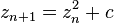# Patterns

This piece is called Looking Through.“Looking Through”
Digital Art printed on metal, single edition
20×20″
\$345.00 Artist: Lianne Todd

Looking through what?  A microscope?  A telescope? A porthole?

In a fractal universe, it doesn’t really matter.  Similar patterns are present on multiple scales.  Use your imagination!

This image is actually a combination of fractals – one for the thing we are looking through (the self-similarity on smaller scales provides the illusion of perspective and depth here), and one for what we are looking at (this is a flame fractal – more about them later).

As illustrated here, fractal geometry is quite versatile.  I’ve seen some discussion on ‘true’ fractals versus ‘near’ fractals and I would like to address that here for a moment.  There seems to be an opinion out there that for a fractal to be ‘true’ it must be a)infinite and b)exactly the same no matter what scale you look at.  Having read most of Benoit Mandelbrot’s Fractal Geometry of Nature, I have a problem with these stipulations.  First of all, the equation for Mandelbrot’s set is$z_{n+1}=z_n^2+c$, with as the number of iterations, where c is a complex parameter.

There is more to explaining the Mandelbrot set than that, of course, but that is the equation, and if n is a given number, then it’s not infinite, is it?  Perhaps the possibility of an infinite number of iterations exists, but that’s an argument for another day.

And even Mandelbrot’s set is not exactly the same on multiple scales. The PATTERN is there, it’s just slightly altered at different scales.  It is self-similar.  This is one of the things which makes fractal geometry so suitable for modelling the universe.

In my understanding, there was never a suggestion by Mandelbrot, the founder of fractal geometry, that a “true” fractal had to be infinite OR exactly the same on multiple scales.  Rather, a fractal is strictly defined as “a set for which the Hausdorff Besicovitch dimension strictly exceeds the topological dimension.”

So, perhaps I’m getting it all wrong, but if you would like to argue I would welcome your discussion.

And now, because I was once a biologist and if you’re anything like me you need a more highly magnified look at that thing, here is a zoom of what you were “looking through” at: